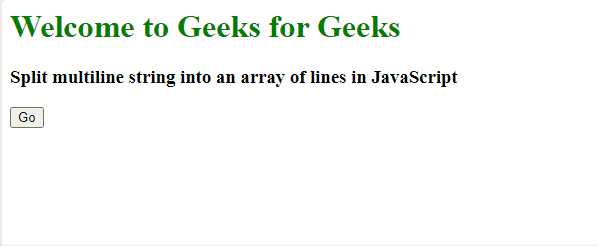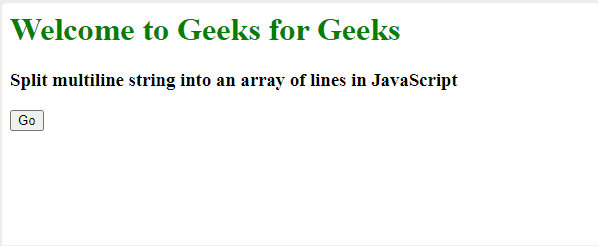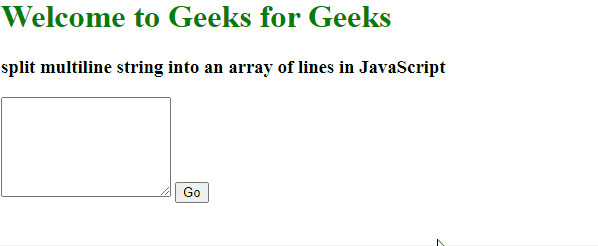Open in App
Not now

# How to split multiline string into an array of lines in JavaScript ?

• Difficulty Level : Easy
• Last Updated : 05 Jan, 2023

Multiline string in JavaScript means a string having two or more lines. To split a multiline string into an array we need to use split() in our JavaScript code.

JavaScript split(separator, limit) Method: The split() function is used to split the data depending upon the attributes we are passing in it. The separator attributes specify that from this word/sign the string will be divided. The limit attribute is optional, it specifies how many splits will be there.

Example 1: This example shows the use of the above approach. In this, when we will click on the “Go” button the array of the multiline string will be displayed on the screen separated by “,”.

## HTML

 `<``h1` `style``=``"color:green"``>` `    ``Welcome to Geeks for Geeks` `` `<``h3``>` `    ``Split multiline string into an array of lines in JavaScript` `` `<``button` `onclick``=``"myFunction()"``>` `    ``Go` ``   `<``p` `id``=``"StringToArray"``>`   `<``script``>` `    ``function myFunction() {` `        ``var string = "Are you ready?" ` `            ``+ "<``br``>So let's get started";` `    `  `        ``var array = string.split("<``br``>");` `    `  `        ``document.getElementById("StringToArray")` `            ``.innerHTML = array;` `    ``}` ``

Output:Split multiline string into an array of lines

Example 2: Now, let’s see how to get a particular index of an array.

## HTML

 `<``h1` `style``=``"color:green"``>` `    ``Welcome to Geeks for Geeks` `` `<``h3``>` `    ``Split multiline string into an array of lines in JavaScript` ``   `<``button` `onclick``=``"myFunction()"``>Go`   `<``p` `id``=``"StringToArray"``>`   `<``script``>` `    ``function myFunction() {` `        ``var string = "Are you ready?" ` `            ``+ "<``br``>So let's get started";` `    `  `        ``var array = string.split("<``br``>");` `    `  `        ``document.getElementById("StringToArray")` `            ``.innerHTML = array;` `    ``}` ``

Output:Split multiline string into an array of lines

As we wrote array, hence only the 2nd line has been printed. If we will write array, then it will be undefined as this array contains data in the first two indexes only that are 0 and 1 respectively.

Example 3: Now, let’s try to take a string as user input.

## HTML

 `<``h1` `style``=``"color:green"``>` `    ``Welcome to Geeks for Geeks` `` `<``h3``>` `    ``split multiline string into an` `    ``array of lines in JavaScript` ``   `<``textarea` `id``=``"write"` `placeholder``=``"Write something"` `          ``style``=``"height:100px;"``>` ``   `<``button` `onclick``=``"myFunction()"``>Go`   `<``p` `id``=``"StringToArray"``>`     `<``script``>` `    ``function myFunction() {` `    `  `        ``var string = document` `            ``.getElementById("write").value;` `    `  `        ``var array = string.split(".");` `    `  `        ``document.getElementById("StringToArray")` `            ``.innerHTML = array;` `    ``}` ``

Output:Split multiline string into an array of lines

My Personal Notes arrow_drop_up
Related Articles